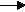gcsescience.com                                       25                                       gcsescience.com

Moles

Masses or Volumes from Electrolysis.

Example 2.

The electrolysis of sodium chloride dissolved in water
produces hydrogen at the cathode and chlorine at the anode.

What mass and volume of hydrogen is obtained
if 142 g  of chlorine are discharged at the anode?

Method.
1) Find how many moles of chlorine are discharged at the anode.

RMM of Cl2 = 71.

moles = mass ÷ RMM

moles = 142 ÷ 71

= 2 moles of chlorine.

2) Write the half equations for the electrolysis.
(i)  2H+  +    2e-H2
(ii)  2Cl-   -    2e-Cl2

Balance the half equations,
to give the same number of electrons on each side.

Find the proportion of how many moles of product
are discharged at each electrode.
1 mole of H2 at the cathode gives 1 mole of Cl2 at the anode.
The proportion is 1 to 1.

2 moles of Cl2 gives 2 moles of H2.

3)  Find the mass and volume of 2 moles of hydrogen.
The RMM of hydrogen is 2.

mass = moles x RMM

mass = 2 x 2
= 4 grams of
hydrogen.

volume moles x 24,000 cm3

volume 2 x 24,000 cm3
= 48,000 cm3 of
hydrogen.

This occupies the same volume as 142  g  of Cl2.

gcsescience.com       The Periodic Table       Index       Moles Quiz       gcsescience.com